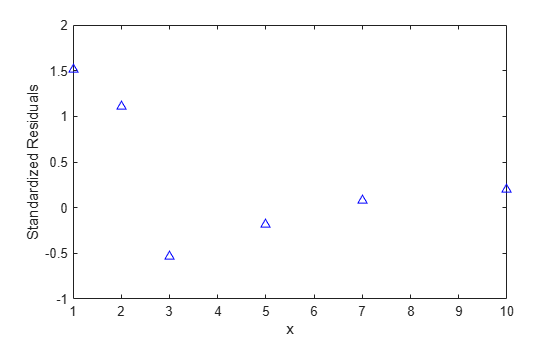# 加权非线性回归

### 要拟合的数据和模型

```x = [1 2 3 5 7 10]'; y = [109 149 149 191 213 224]'; plot(x,y,'ko') xlabel('Incubation (days), x') ylabel('Biochemical oxygen demand (mg/l), y')```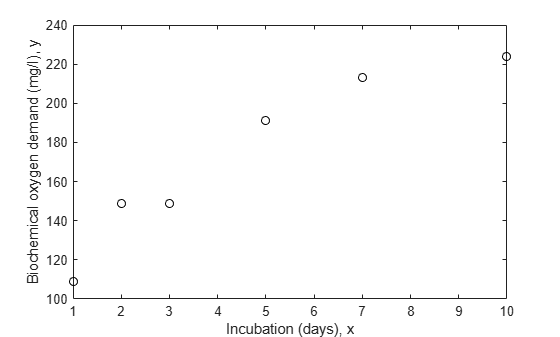`w = [1 1 5 5 5 5]';`

### 不加权拟合模型

`modelFun = @(b,x) b(1).*(1-exp(-b(2).*x));`

`start = [240; .5];`

```nlm = fitnlm(x,y,modelFun,start); xx = linspace(0,12)'; line(xx,predict(nlm,xx),'linestyle','--','color','k')```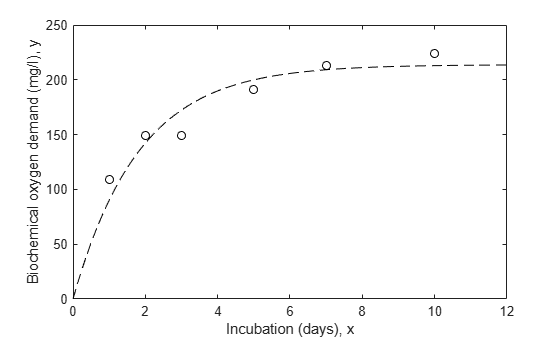### 加权拟合模型

`wnlm = fitnlm(x,y,modelFun,start,'Weight',w)`
```wnlm = Nonlinear regression model: y ~ b1*(1 - exp( - b2*x)) Estimated Coefficients: Estimate SE tStat pValue ________ ________ ______ __________ b1 225.17 10.7 21.045 3.0134e-05 b2 0.40078 0.064296 6.2333 0.0033745 Number of observations: 6, Error degrees of freedom: 4 Root Mean Squared Error: 24 R-Squared: 0.908, Adjusted R-Squared 0.885 F-statistic vs. zero model: 696, p-value = 8.2e-06 ```
`line(xx,predict(wnlm,xx),'color','b')`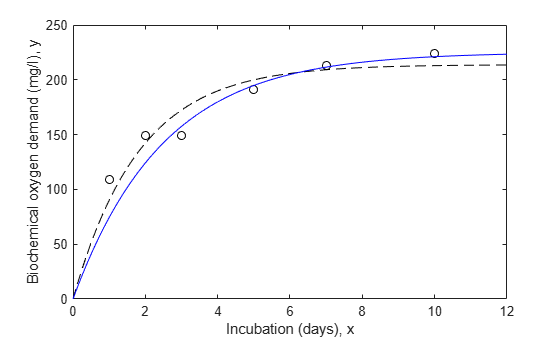`wnlm.RMSE`
```ans = 24.0096 ```

`coefCI(wnlm)`
```ans = 2×2 195.4650 254.8788 0.2223 0.5793 ```

### 估计响应曲线

```[ypred,ypredci] = predict(wnlm,xx,'Simultaneous',true); plot(x,y,'ko',xx,ypred,'b-',xx,ypredci,'r:') xlabel('x') ylabel('y') ylim([-150 350]) legend({'Data','Weighted fit','95% Confidence Limits'}, ... 'location','SouthEast')```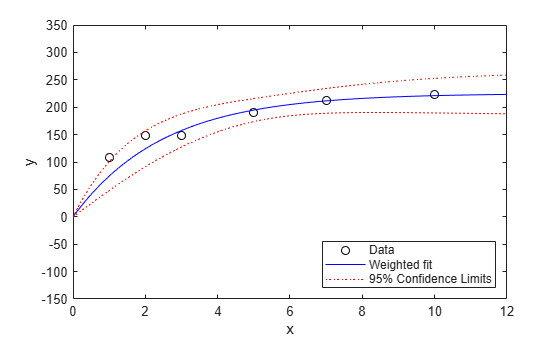```[ypred,ypredci] = predict(wnlm,xx,'Simultaneous',true, ... 'Prediction','observation'); plot(x,y,'ko',xx,ypred,'b-',xx,ypredci,'r:') xlabel('x') ylabel('y') ylim([-150 350]) legend({'Data','Weighted fit','95% Prediction Limits'}, ... 'location','SouthEast')```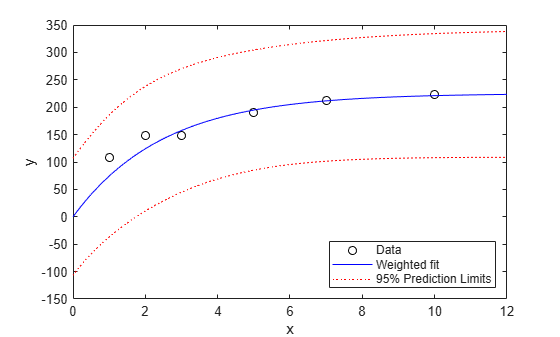```[new_ypred,new_ypredci] = predict(wnlm,xx,'Simultaneous',true, ... 'Prediction','observation','Weights',5*ones(size(xx))); plot(x,y,'ko',xx,new_ypred,'b-',xx,new_ypredci,'r:') xlabel('x') ylabel('y') ylim([-150 350]) legend({'Data','Weighted fit','95% Prediction Limits'}, ... 'location','SouthEast')```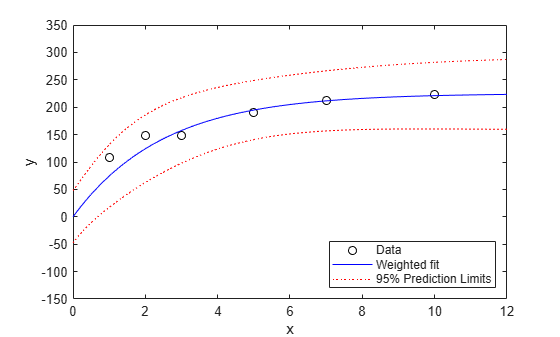`predict` 函数通过 `MSE*(1/W(i))` 估计观测值 `i` 处的误差方差，其中 `MSE` 是均方误差。因此，置信区间变窄。

### 残差分析

```r = wnlm.Residuals.Standardized; plot(x,r,'b^') xlabel('x') ylabel('Standardized Residuals')```